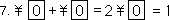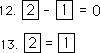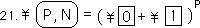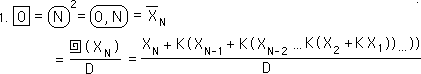## 9.2 Pascal's ∆ & a Binomial Expansion

### A. A Binomial Diversion: Internal & External Operations in the Seed Equation

#### Another Version of the Seed Equation, the N-Square

Before proceeding on, a brief diversion:

As scientists say, "Where there is a Pascal's Triangle, there is a Binomial Expansion, lurking somewhere around." Remember that the Seed Equation, when expanded had Binomial Coefficients, Pascal's Triangle. We never explored its elements. Below is another way of expressing the Seed Equation. This reveals a different side to the underlying structure of a seed.#### Demonstration

Below is a brief demonstration of how this Binomial Expansion works. We derive the expected result.#### Moments of Amazement: Internal & External Operations

The N-Square operation behaves just like simple addition, internally. Addition is still employed externally in the combination of like terms. But square terms when ‘multiplied’ times each other are added internally. When a square term is raised to a power it is like multiplication, internally. The elements of a Directional employ both internal and external addition in the expansion from seed form. The internal addition is like external multiplication in that it is distributed across external addition the same way, i.e. the Distributive Law applies. We can even raise our square terms to powers, but because multiplication is internal addition, raising to powers is internal multiplication. We call these internal because the changes occur inside the square. The external changes occur outside. External multiplication is internal addition. External powers are internal multiplication. If the reader is not thoroughly confused then he is alone in this world. The bottom notational definition illustrates how external multiplication turns into internal addition.#### A second level of amazement: or many amazing questions

How does this system get away with calling multiple Unravelings unitary? How is it that a scaled average of the Now, R=0, can be called '0'? How can the scaled average of this average, once removed from the Now, a triple unfurling of the once past data divided by D squared, be called '1'. How can these two diverse Unravelings be added together internally, 0+1=1, multiplied externally, to get another internal '1'. Why are these internal integers? And how do they relate with external integers? Finally how can these unitary expressions of the Unravelings be multiplied externally to yield all the Directionals? How many ways can you amaze us, Mom, Mommy, Mother Nature, the Source? We bow down before your majesty, complexity and simplicity, complicity and simplexity.

#### The Box

Our box that holds these unitary Ravelings is very tight. It doesn't hold all the Ravelings. It only holds one in each category of Raveling, one and only one. Not two, not none, not many, just one. Not all possibilities, only one narrow path. The power of the Unraveling is determined by how many times it is removed from the Now and vice versa. In other words, a double Unraveling only applies to the Now. A triple Unraveling only applies to the spot once removed from the Now. A quadruple Unraveling only applies to the spot twice removed from the Now. And so on. A tight box, no leeway.

### B. The Goal: Potential Impact of Data upon Derivatives

#### We are poised and ready

We've positioned ourselves and we're ready. We've now reached the point where we can start looking at the potential impact of the Data upon the derivatives themselves. We know from previous derivations that the derivatives are a function of Raveled Data, {See The Seed Equation Notebook.} We know the potential impact of the individual data points upon the multiple Unravelings. How are they mixed?

#### Going in for the kill

The 1st Directional equals the difference between zero-square and one-square.The 1st Directional is a measure that represents the average amount of change. When there is no change the 1st Directional must equal zero. Let us imagine a living Data Stream that dies. Our stream ends with an infinite string of zero data points. (Our Stream has not been killed. It has not been functionalized.) All the derivatives would approach zero. We will say that this Stream is approaching equilibrium.At the point of equilibrium, when there is no change, zero-square must equal one-square. Hence the potential impact of zero-square must be equal to one-square because they have an equal and opposite effect upon the 1st Directional.The potential impact does not change. If the potential impact of zero-square equals one-square, then they are always equal. The total impact is equal to the sum of the individual impacts and is always equal to one, one hundred percent.The potential impacts of zero-square and one-square are equal. We substitute for one-square.Solving the equation we find that the potential impact of both, zero-square and one-square, equal one half of the potential impact upon the 1st Directional.Thus to find the potential impact of any individual Data Point upon the first Directional, one would need to find the sum of the potential impact of the Data upon zero-square and then on one-square and halve it.#### The 2nd Directional, Potential Impact, potential impact

The 2nd Directional in square language is written as follows.As our Stream dies, approaches equilibrium, the 2nd Directional approaches zero.Therefore the sum of the square numbers, which yield the 2nd Directional, would also equal zero.We already know that the difference of zero-square and one-square approaches zero at equilibrium. Reducing our equation, we find that two-square approaches/equals one-square as our system approaches equilibrium.Using the same reasoning as with the 1st Directional: If they approach each other when the Stream approaches equilibrium then their potential impact of both halves must also be equal. Because, the potential impact, the potential impact, of two-square equals one-square, which we already know is also equal to zero-square.The potential impact does not change; hence the potential impact of the 3 squares remains equal. The total potential impact equals the sum of the individual potential impacts. The individual squares have the same potential impact. Hence we substitute zero-square's potential impact for the others. Solving our equation algebraically we find that each square is one fourth of the total potential impact of the 2nd Directional. One-square is used twice; hence its potential impact on the total is doubled to one half.To determine the impact of an individual piece of Data upon the 2nd Directional one would have to use the equation below.#### General Potential Impact Equation for the Directionals

For the general Directional the same reasoning is used as on the specific. The total potential impact is always one.Remembering the general square equation for the Directionals, we see that the whole shebang is built around zero-square and one-square at different levels.When the Stream approaches equilibrium, dies, the derivatives approach zero, hence all the parts approach zero and are equal. Hence their potential impacts are also equal.The total sum of the individual impacts equals the total impact. Thus, we can write the general potential impact for Directionals as follows. The sum of the coefficients in a binomial expansion equals 2P; another way of saying this is that the number of elements in the uncombined expansion is 2P. Because each of the potential impacts is equal, each individual element has a 1/2P potential impact on the whole. Some individual squares are used more than once in the expansion; hence their overall impact is multiplied by the number of times they are used.The potential impact function is separate from the interaction of the squares. The expansion is the same with or without the potential impact sign, because the total potential impact is still the sum of the individual potential impacts and the binomial expansion is ultimately a sum. Below is shown the mechanism.The potential impact function is also independent of the individual potential impacts impact upon the whole. This is seen in the notation below. Remember that in these binomial expansions that '*' is only an operator symbol. It does not represent traditional multiplication. (We will speak of this soon.)We finally reach our operating equation for the potential impact of an individual piece of data upon any Directional. This is the equation that was used to generate the potential impacts of the individual data for the Directionals for the preceding graphs.#### Experimental verification

As R begins at zero and goes higher and higher, the sum of the individual potential impacts approaches one. This has been confirmed from the zeroth to the 3rd Directional, when P=0->3. Since the 3rd Directional is the sum and differences of Ravelings from zero to three, it also is a sub-confirmation of the accuracy of their equations and the reasoning above. (That the sum of the series for the Ravelings, 0->3, approaches one, has already been confirmed independently.)

### C. Soon: Operators & Operators

#### Laws & Operators in the Binomial Expansion

The operator, *, in our square Seed-Equations above is not multiplication in the traditional sense. It is an operator in the sense that it fulfills the obligations of a Binomial Expansion. In this application, the coefficients and elements turn out right. We don't know why. We don't know what the operation is. Below is a brief diagram of the elements of a simple Binomial Expansion. It shows which laws are operating in the generation of the coefficients. We assume that '+' means addition and '*' means multiplication in the traditional senses. However any system, which contains operators and operands that behave according to the equations below, must also obey its laws.#### The Seed Equation & the Binomial Expansion

We have inadvertently found a system that generates coefficients and operators in the manner of a Binomial Expansion. We know that traditional multiplication is defined as a series of additions, while additions are a series of counting. While the addition operator, '+', behaves identically in this Derivative system, the multiplication operator, '*', behaves much differently. Also X and Y do not represent traditional numbers. They represent numbers, which have been raveled one or more times. These numbers are elements of a Data Stream and raveled over all the past numbers of the Stream. Below is a binomial expansion of our system on the simplest level.

#### A Review of Square NotationNote that the Distributive Law and the Commutative Law are both applied in the expansion. Now let us look at what zero-square, one-square, and two-square represent. They certainly behave like normal numbers in this expansion. Below is zero-square unraveled and unfurled, not quite such a normal number after all.

#### Unraveled and Unfurled, the SquaresBelow is one-square, made up of scaled zero-squares, a triple unraveling, a double unfurling of XN-1.Below is two-square, made up of scaled one-squares, a quadruple unraveling, a triple unfurling of XN-2. Although they look and behave like normal numbers, they are not normal and are actually incredibly complex, even at this elementary level.#### We give. What's the operator?

Remember these three operations. Zero-square times zero-square equals zero-square. Makes sense until you look at zero-square, above. Zero-square times one-square equals one-square. Sounds a little like addition until you refer to zero-square and one square above. Then it becomes apparent that the XN term disappears entirely in the multiplication, the operation, '*'. Finally one-square times one-square equals two-square. Seems like a type of addition until focusing upon one-square and realizing that in the squaring of itself it loses its XN-1 term and gains an incredible complexity.We don't know what our operator means in algebraic terms, haven't the foggiest. Geometrically, we conceive of zero-square as the Now. One-square is one dimension extra, one time period away from Now. Two-square is two-dimensions greater than zero-square and separated by to time periods from Now. When one-square operates on another square it tells it to add a dimension and a level of separation from Now. Zero-square says stay the same. Two-square says to add two dimensions and two levels of separation. We don't know why. You tell us.